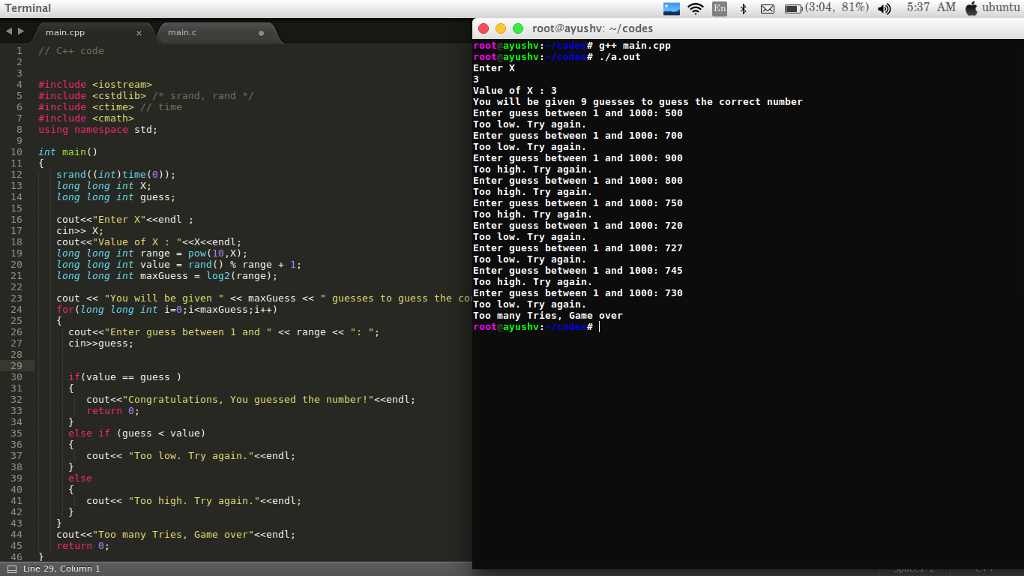# Answered! I need help making C++ programming. Code and flowchart….

```I need help making C++ programming. Code and flowchart.

Write a program that plays the game "Guess the Number" as follows.  Your program chooses the number to be guessed by selecting an integer at random in the range 1 to 10^X.  The program inputs X and displays.

I have a number between 1 and 10^X Can you guess my number?  You will be given a maximum of log2(10^X)+1 guesses.

The player then types a guess.  The program responds with one of the following.

1. Congratulations, You guessed the number! Would you like to play again(y or n)?
2. Too low.  Try again.
3. Too High. Try again.
4. Too many tries.
```
```Flowchart the results.
```

// C++ code

#include <iostream>
#include <cstdlib> /* srand, rand */
#include <ctime> // time
#include <cmath>
using namespace std;

int main()
{
srand((int)time(0));
long long int X;
long long int guess;

Don't use plagiarized sources. Get Your Custom Essay on
Answered! I need help making C++ programming. Code and flowchart….
GET AN ESSAY WRITTEN FOR YOU FROM AS LOW AS \$13/PAGE

cout<<“Enter X”<<endl ;
cin>> X;
cout<<“Value of X : “<<X<<endl;
long long int range = pow(10,X);
long long int value = rand() % range + 1;
long long int maxGuess = log2(range);

cout << “You will be given ” << maxGuess << ” guesses to guess the correct numbern”;
for(long long int i=0;i<maxGuess;i++)
{
cout<<“Enter guess between 1 and ” << range << “: “;
cin>>guess;

if(value == guess )
{
cout<<“Congratulations, You guessed the number!”<<endl;
return 0;
}
else if (guess < value)
{
cout<< “Too low. Try again.”<<endl;
}
else
{
cout<< “Too high. Try again.”<<endl;
}
}
cout<<“Too many Tries, Game over”<<endl;

return 0;
}